Quantitative MCQ - 14

# Quantitative MCQ - 14

Test Description

## 30 Questions MCQ Test Quantitative Aptitude for Competitive Examinations | Quantitative MCQ - 14

Quantitative MCQ - 14 for Quant 2023 is part of Quantitative Aptitude for Competitive Examinations preparation. The Quantitative MCQ - 14 questions and answers have been prepared according to the Quant exam syllabus.The Quantitative MCQ - 14 MCQs are made for Quant 2023 Exam. Find important definitions, questions, notes, meanings, examples, exercises, MCQs and online tests for Quantitative MCQ - 14 below.
Solutions of Quantitative MCQ - 14 questions in English are available as part of our Quantitative Aptitude for Competitive Examinations for Quant & Quantitative MCQ - 14 solutions in Hindi for Quantitative Aptitude for Competitive Examinations course. Download more important topics, notes, lectures and mock test series for Quant Exam by signing up for free. Attempt Quantitative MCQ - 14 | 30 questions in 30 minutes | Mock test for Quant preparation | Free important questions MCQ to study Quantitative Aptitude for Competitive Examinations for Quant Exam | Download free PDF with solutions
 1 Crore+ students have signed up on EduRev. Have you?
Quantitative MCQ - 14 - Question 1

### Directions (1-5): Production of three different flavors of Soft-Drinks Pepsi, Thumbs Up & Sprite by Pepsico. Over three years (in lakhs)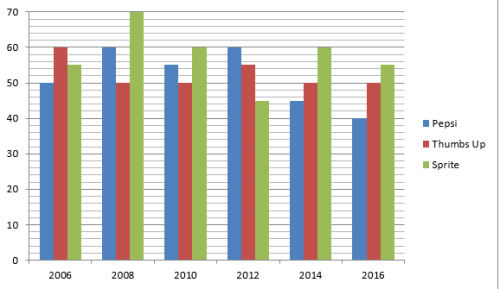For which of the following years, the % of rise or fall in production from previous years is maximum for Sprite?

Detailed Solution for Quantitative MCQ - 14 - Question 1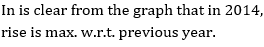Quantitative MCQ - 14 - Question 2

### Directions (1-5): Production of three different flavors of Soft-Drinks Pepsi, Thumbs Up & Sprite by Pepsico. Over three years (in lakhs)For which flavor was the average production maximum in given period?

Detailed Solution for Quantitative MCQ - 14 - Question 2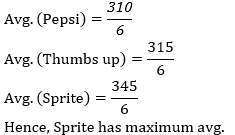Quantitative MCQ - 14 - Question 3

### Directions (1-5): Production of three different flavors of Soft-Drinks Pepsi, Thumbs Up & Sprite by Pepsico. Over three years (in lakhs)Total production for Pepsi in 2010 & 2012 is what % of the total production of Thumbs Up in 2014 & 2010 together?

Detailed Solution for Quantitative MCQ - 14 - Question 3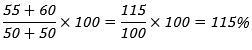Quantitative MCQ - 14 - Question 4

Directions (1-5): Production of three different flavors of Soft-Drinks Pepsi, Thumbs Up & Sprite by Pepsico. Over three years (in lakhs)What is the difference between the average production of Sprite in 2006, 2010 & 2016 and the average production of Sprite in 2008, 2010 &2014?

Detailed Solution for Quantitative MCQ - 14 - Question 4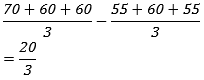Quantitative MCQ - 14 - Question 5

Directions (1-5): Production of three different flavors of Soft-Drinks Pepsi, Thumbs Up & Sprite by Pepsico. Over three years (in lakhs)What was the approximate decline in the production of Thumbs Up in 2016 from 2006?

Detailed Solution for Quantitative MCQ - 14 - Question 5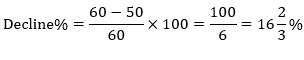Quantitative MCQ - 14 - Question 6

Directions (6-10): In the following questions, two equations numbered I and II are given. You have to solve both the equations

I. x2+13x+42=0

II. y2+19y+90=0

Detailed Solution for Quantitative MCQ - 14 - Question 6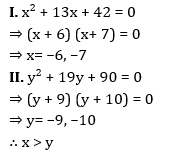Quantitative MCQ - 14 - Question 7

Directions (6-10): In the following questions, two equations numbered I and II are given. You have to solve both the equations

I. x2+15x+56=0

II. y2-23y+132=0

Detailed Solution for Quantitative MCQ - 14 - Question 7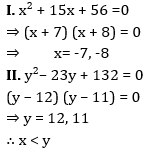Quantitative MCQ - 14 - Question 8

Directions (6-10): In the following questions, two equations numbered I and II are given. You have to solve both the equations

I. x2=144

II. y2-24y+144=0

Detailed Solution for Quantitative MCQ - 14 - Question 8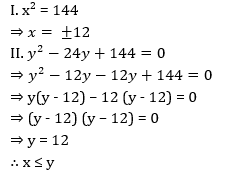Quantitative MCQ - 14 - Question 9

Directions (6-10): In the following questions, two equations numbered I and II are given. You have to solve both the equations

I. 2x2+19x+45=0

II. 2y2+11y+12=0

Detailed Solution for Quantitative MCQ - 14 - Question 9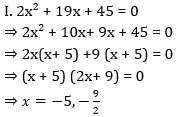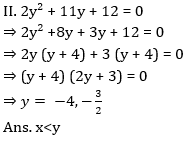Quantitative MCQ - 14 - Question 10

Directions (6-10): In the following questions, two equations numbered I and II are given. You have to solve both the equations

I. 3x2-13x+12=0

II. 2y2-15y+28=0

Detailed Solution for Quantitative MCQ - 14 - Question 10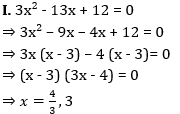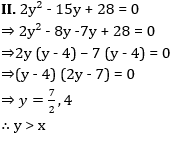Quantitative MCQ - 14 - Question 11

Direction (11-15): Refer the following line graph and answer the questions based on it.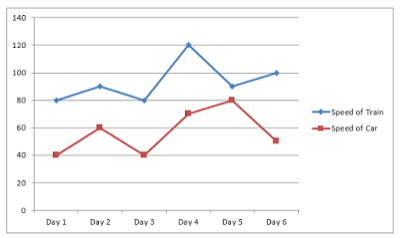What is the average speed of the train on Day 1, Day 3 and Day 4 together if the train travelled same distance on each of these days?

Detailed Solution for Quantitative MCQ - 14 - Question 11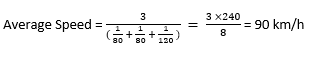Quantitative MCQ - 14 - Question 12

Direction (11-15): Refer the following line graph and answer the questions based on it.On day 5, the train covered a distance of 300 km while the car covered 240 km. What is the ratio of time taken by the car to the time taken by the train on that day?

Detailed Solution for Quantitative MCQ - 14 - Question 12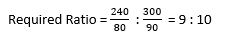Quantitative MCQ - 14 - Question 13

Direction (11-15): Refer the following line graph and answer the questions based on it.If the time taken by the car is thrice to the time taken by the train on day 3, then the distance covered by the car is how much percent more than the distance covered by the train on that day?

Detailed Solution for Quantitative MCQ - 14 - Question 13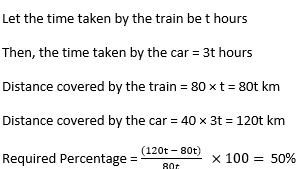Quantitative MCQ - 14 - Question 14

Direction (11-15): Refer the following line graph and answer the questions based on it.For which day, the percentage increase/decrease in the speed of train from the previous day is the maximum?

Detailed Solution for Quantitative MCQ - 14 - Question 14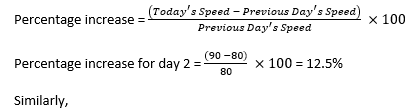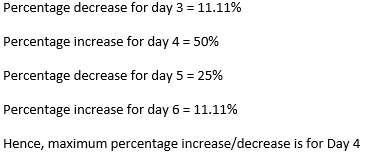Quantitative MCQ - 14 - Question 15

Direction (11-15): Refer the following line graph and answer the questions based on it.If both the train and the car travelled for 3 hours 20 minutes each on day 2, then what is the difference of the distances travelled by the train and the car on that day?

Detailed Solution for Quantitative MCQ - 14 - Question 15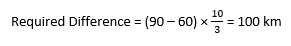Quantitative MCQ - 14 - Question 16

Directions (16-19): Study the following pie chart and answer the questions based on it.

Given below is the pie chart which shows the percentage of female voters in 5 different villages.
Total female voters in these five villages is 12000.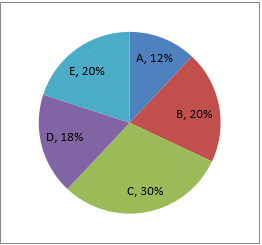What is the sum of male voters from village A and B together if total voters (Male + Female) in these two villages in 7600

Detailed Solution for Quantitative MCQ - 14 - Question 16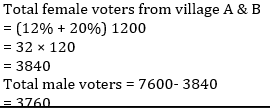Quantitative MCQ - 14 - Question 17

Directions (16-19): Study the following pie chart and answer the questions based on it.

Given below is the pie chart which shows the percentage of female voters in 5 different villages.
Total female voters in these five villages is 12000.What is the ratio of total female voters from village C & D together to the total female voters from village B and E together

Detailed Solution for Quantitative MCQ - 14 - Question 17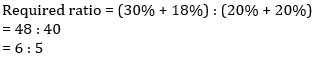Quantitative MCQ - 14 - Question 18

Directions (16-19): Study the following pie chart and answer the questions based on it.

Given below is the pie chart which shows the percentage of female voters in 5 different villages.
Total female voters in these five villages is 12000.Total female voters from village A and C together are what percent of female voters village D and E together.

Detailed Solution for Quantitative MCQ - 14 - Question 18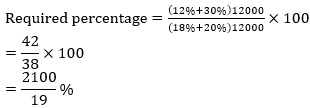Quantitative MCQ - 14 - Question 19

Directions (16-19): Study the following pie chart and answer the questions based on it.

Given below is the pie chart which shows the percentage of female voters in 5 different villages.
Total female voters in these five villages is 12000.If ratio of male to female voters from village C is 6 : 5 and from village D is 4 : 3 then males from village C are what percent of males from  village D.

Quantitative MCQ - 14 - Question 20

Directions (20-24): In the following number series only one number is wrong. Find out the wrong number.

3     7     16     35     72     153     312

Detailed Solution for Quantitative MCQ - 14 - Question 20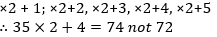Quantitative MCQ - 14 - Question 21

Directions (20-24): In the following number series only one number is wrong. Find out the wrong number.

18     20     23     32     48     73     109

Detailed Solution for Quantitative MCQ - 14 - Question 21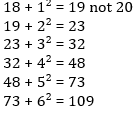Quantitative MCQ - 14 - Question 22

Directions (20-24): In the following number series only one number is wrong. Find out the wrong number.

7     4     5     9     20     51     160.5

Detailed Solution for Quantitative MCQ - 14 - Question 22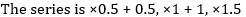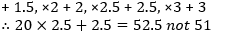Quantitative MCQ - 14 - Question 23

Directions (20-24): In the following number series only one number is wrong. Find out the wrong number.

6     10     14     34     66     130     258

Detailed Solution for Quantitative MCQ - 14 - Question 23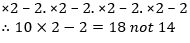Quantitative MCQ - 14 - Question 24

Directions (20-24): In the following number series only one number is wrong. Find out the wrong number.

2     7     30     138     524     1557     3102

Detailed Solution for Quantitative MCQ - 14 - Question 24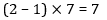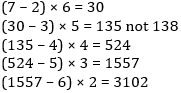Quantitative MCQ - 14 - Question 25

Directions (25–29): Study the following pie-charts carefully and answer the questions below it.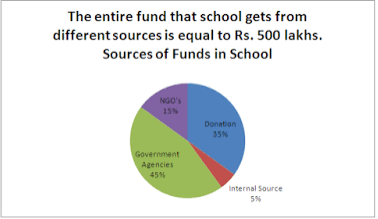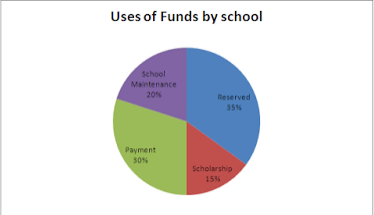What is the difference between the funds acquired by school from NGO’s and internal sources?

Detailed Solution for Quantitative MCQ - 14 - Question 25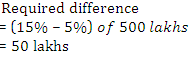Quantitative MCQ - 14 - Question 26

Directions (25–29): Study the following pie-charts carefully and answer the questions below it.If the school managed ‘school maintenance’ from the ‘government agencies’ only, then how much fund from government agencies would still be left for other use?

Detailed Solution for Quantitative MCQ - 14 - Question 26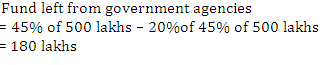Quantitative MCQ - 14 - Question 27

Directions (25–29): Study the following pie-charts carefully and answer the questions below it.If scholarship has to be paid out of the donation found, the what is the approximate per cent of donation found used for this purpose?

Detailed Solution for Quantitative MCQ - 14 - Question 27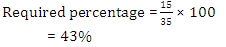Quantitative MCQ - 14 - Question 28

Directions (25–29): Study the following pie-charts carefully and answer the questions below it.What is the total amount used by the school for payment?

Detailed Solution for Quantitative MCQ - 14 - Question 28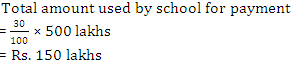Quantitative MCQ - 14 - Question 29

Directions (25–29): Study the following pie-charts carefully and answer the questions below it.What amount of the fund is acquired by the school from government agencies?

Detailed Solution for Quantitative MCQ - 14 - Question 29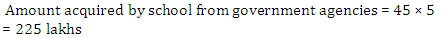Quantitative MCQ - 14 - Question 30

By selling 12 marbles for a rupee, a shopkeeper loses 20% In order to gain 20% in the transaction, he should sell the marbles at the rate of how many marbles for a rupee?

Detailed Solution for Quantitative MCQ - 14 - Question 30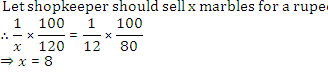## Quantitative Aptitude for Competitive Examinations

35 videos|53 docs|148 tests
 Use Code STAYHOME200 and get INR 200 additional OFF Use Coupon Code
Information about Quantitative MCQ - 14 Page
In this test you can find the Exam questions for Quantitative MCQ - 14 solved & explained in the simplest way possible. Besides giving Questions and answers for Quantitative MCQ - 14, EduRev gives you an ample number of Online tests for practice

## Quantitative Aptitude for Competitive Examinations

35 videos|53 docs|148 tests